# Slice Reconstruction

## Radon Transform

The Radon transform of an image represented by the function f(x,y) can be defined as a series of line integrals through f(x,y) at different offsets from the origin. This is shown in Figure 1 and defined mathematically as: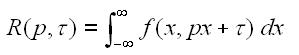where p and tau are the slope and intercepts of the line.

A more directly applicable form of the transform can be defined by using a delta function:where theta is the angle of the line, and r is the perpendicular offset of the line.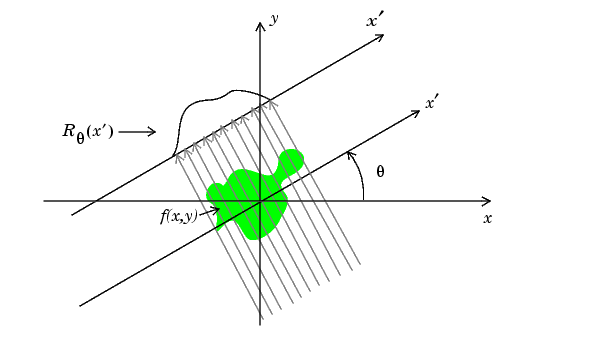Figure 1
Radon Transform

The acquisition of data in medical imaging techniques such as MRI, CT and PET scanners involves a similar method of projecting a beam through an object, and the data is in a similar form to that described in the second equation above. The plot of the Radon transform, or scanner data, is  referred to as a  sinogram due to its characteristic sinusoid shape. Figure 2 shows a simple non-homogeneous shape and the sinogram created by taking the Radon transform at intervals of one degree from 0 to 180 degrees.Figure 2
A simple image (left) and the sinogram (right) produced by applying the Radon Transform.

## Inverse Radon Transform using Filtered Back Projection

To reconstruct the image from the sinogram, the inverse Radon transform is applied to the image. There are several techniques by which the inverse transform can be calculated but the most common is Filtered Back Projection.

The filtered back projection algorithm is split into two phases, filtration and projection.

### Projection

The projection phase is very similar to the Radon transform described above,  and shown in Figure 1 except now the line integrals are projected back onto the plane at their respective angles. The projection phase of the Filtered Back Projection, using the data format described above: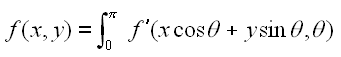where f' is the filtered data.

A common discrete approximation of this is:This equation can be used to determine the pixel values at a given point. The exact values depend on the chosen interpolation method, e.g. nearest-neighbour, linear interpolation etc. As more projections are added, the quality of the reconstruction will increase. Figure 3 shows the box shape reconstructed using 18 projections. This is clearly not enough to accurately reconstruct the image, but the individual projections can be seen.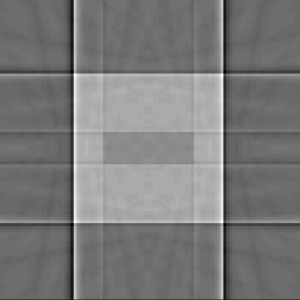Figure 3
Box reconstructed using 18 projections

### Filtration

Figure 4 (left)  shows the result of the back projection algorithm applied as described and using all available data. Although the shape of the reconstructed object can be seen, the reconstructed image is heavily blurred. To counteract this effect, a high pass filter is applied to the sinogram data in the frequency domain.  This is achieved by applying a 1-D DFT to the sinogram data for each angle, multiply by the filter, and then using the inverse DFT to reconstruct the data. The simplest form of high pass filter is a ramp. Applying the ramp filter significantly improves the quality of the reconstructed image, Figure 4 (right). However, because the ramp filter emphasises high frequency components of the image, it can cause unwanted noise. To counteract this, several other high-pass filters are commonly used. A selection of these are shown in Figure 5. See the reference for a full discussion, including derivations, on the use of filtration.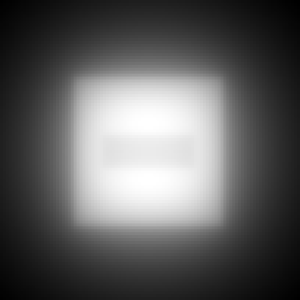Figure 4
Reconstructed images: unfiltered (left) and ramp filtered (right)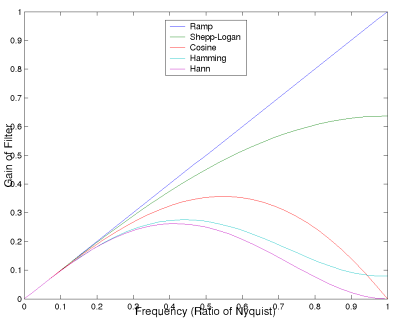Figure 5
Filter windows

As a more realistic demonstration of the back projection algorithm Figure 6 shows a reconstruction based on real medical data. The image on the left is the sinogram obtained from the scanner, the right is a false colour image of the skull reconstructed from this sinogram. The Hann window was used during the filtration step.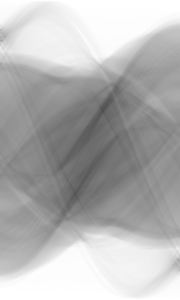Figure 6
Example of filtered back projection applied to medical data.

References:
Peter Toft: "The Radon Transform - Theory and Implementation", Ph.D. thesis. Department of Mathematical Modelling, Technical University of Denmark, June 1996.

Image credits:
Radon transform diagram from MATLAB online documentation http://www.mathworks.com/access/helpdesk/help/toolbox/images/radon.html
Sinogram of skull from http://www-rcp.ijs.si/~zdravkok/int_fbp/int_fbp.htm

Brendan F. Hayden
10/02/2005